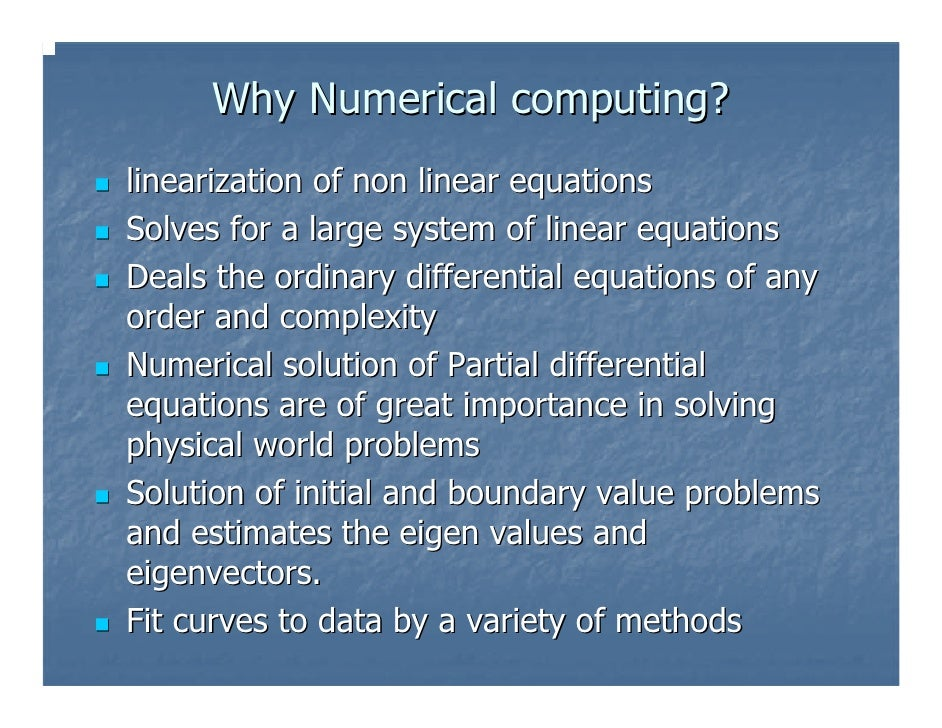# Numerical Computing: Home

In this course emphasis will be laid, on learning the Numerical methods to solve the Linear, Non-linear Equations, Interpolation and Different Numerical Methods to solve the problems of Integration, Differentiation and Differential Equations

## Course Objective

• Grasp the numerical techniques and should be able to use a variety of methods in solving real-life, practical, technical, and theoretical problems which cannot be solved by other methods.
• Apply the Bisection, Regula Falsi, Newton and Iteration methods to solve a non-linear equation
• Apply the different method to solve linear Equations
• Construct Lagrange and Newton forward difference interpolation polynomials for a given set of data.
• Apply Trapezoidal and Simpson’s rules to find the approximate value of an integral.

## Numerical Computing## Deputy Director